﻿ BDS/INS深组合导航仿真平台研究 Study of Simulation Platform for BDS/INS Deep Integration Navigation

Journal of Sensor Technology and Application
Vol. 07  No. 02 ( 2019 ), Article ID: 30013 , 8 pages
10.12677/JSTA.2019.72008

Study of Simulation Platform for BDS/INS Deep Integration Navigation

Tianhu Guo

School of Civil Engineering, Luoyang Institute of Science and Technology, Luoyang Henan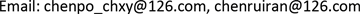Received: Apr. 8th, 2019; accepted: Apr. 22nd, 2019; published: Apr. 29th, 2019ABSTRACT

BDS/INS deep integration navigation systems can improve high precision navigation in the presence of high-dynamics, high-jamming environments, and is the focus of the current research. The design and implementation issues about constructing simulation platform for BDS/INS deep integration navigation are shown. The whole structure and information flow of the simulation platform is presented. The platform is convenient for evaluation and validation of BDS/INS deep integration navigation performance. Simulation shows that the deep integration system can improve the navigation accuracy greatly compared with tight integration system, with the position error less than 4 m and velocity error less than 0.2 m/s.

Keywords:BDS/INS, Deep Integration, Simulation Platform, Performance Analysis

BDS/INS深组合导航仿真平台研究BDS/INS深组合导航可以提高系统的导航精度、抗干扰性和动态性能，是目前研究的重点和热点。本文给出了BDS/INS深组合导航仿真平台的设计及实现，介绍了仿真平台的总体体系结构、信息流程及各分系统的组成。该平台便于进行深组合导航系统算法及性能的验证分析，仿真验证结果表明，在高动态条件下，BDS/INS深组合导航系统的位置误差稳定在4 m范围内，速度误差稳定在0.2 m/s内。Copyright © 2019 by author(s) and Hans Publishers Inc.

This work is licensed under the Creative Commons Attribution International License (CC BY).

http://creativecommons.org/licenses/by/4.0/1. 引言

GNSS (Global Navigation Satellite System)和INS (Inertial Navigation System)具有良好的优势互补性，因此GNSS/INS组合导航被业内称之为“黄金组合”    ，根据观测量的不同，有松组合、紧组合和深组合三种组合模式    。GNSS/INS深组合是一种深层次的、基于硬件层面的组合方式，实现了INS导航参数与GNSS接收机相关器输出的I/Q信号的融合，在高动态、强干扰、弱信号及遮挡环境中具有优越的导航性能，是今后GNSS/INS组合导航应用的主要发展方向。目前，我国的北斗卫星导航系统(BeiDou Navigation Satellite System, BDS)已经在亚太大部分区域得到了广泛的应用，BDS/INS深组合导航系统在民用领域可应用于无人驾驶、精密精近飞机着陆、测绘、地图绘制等，在军用领域可应用于导弹、制导炸弹、单兵导航、无人侦察机等。由于其优越的性能及广泛的应用前景，得到国内外相关大学、研究机构高度重视  -  。

BDS/INS深组合导航是INS信息与BDS接收机跟踪层面的融合，涉及到BDS接收机内部编排，在高动态、强干扰应用环境下进行物理或半物理仿真试验难度大，考虑因素较多   。而构建BDS/INS深组合导航仿真平台可以建立和提供接近实际的物理模型和工作参数，从而为方案论证提供依据，经济性和适应性强  。参照GSNRx-utTM设计思想，本文构建了BDS/INS深组合导航仿真平台，给出了仿真平台的体系结构、信息流程及各分系统的组成，该仿真平台可以同步输出BDS中频数据、INS观测数据，具备基带信号处理功能、INS导航解算功能、组合导航滤波解算等功能，可用于对BDS/INS深组合导航系统在高动态和弱信号环境下的导航性能进行仿真分析。此平台预留接口，可以扩展到其它GNSS/INS深组合导航系统。

2. 深组合导航系统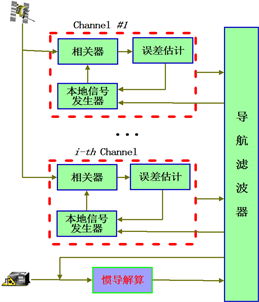Figure 1. BDS/INS deep combined structure

3. BDS/INS深组合导航仿真平台

BDS/INS深组合导航仿真平台主要由以下几部分构成：运动轨迹发生器，BDS中频信号仿真器，BDS接收机基带信号处理，IMU信号产生器，INS力学编排、组合导航滤波器。平台总体结构如图2所示。Figure 2. Overall structure of the deep combined simulation platform

3.1. 运动轨迹发生器

3.2. IMU信号产生器

①陀螺仪仿真器

${\stackrel{˜}{\omega }}_{ib}^{b}={\omega }_{ib}^{b}+{\epsilon }_{g}^{b}$ (1)

${\omega }_{ib}^{b}={R}_{L}^{b}\left({\omega }_{ie}^{L}+{\omega }_{eL}^{L}\right)+{\omega }_{Lb}^{b}$ (2)

${\omega }_{Lb}^{b}=\left[\begin{array}{ccc}\mathrm{cos}r& 0& \mathrm{cos}p\mathrm{sin}r\\ 0& 1& \mathrm{sin}p\\ \mathrm{sin}r& 0& \mathrm{cos}p\mathrm{cos}r\end{array}\right]\cdot \left[\begin{array}{c}\stackrel{˙}{p}\\ \stackrel{˙}{r}\\ \stackrel{˙}{y}\end{array}\right]$ (3)

②加速度计仿真器

${\stackrel{˜}{f}}^{b}={f}^{b}+{\nabla }_{a}^{b}$ (4)

${f}^{b}={R}_{L}^{b}\left[{\stackrel{˙}{V}}^{L}+\left(2{\Omega }_{ie}^{L}+{\Omega }_{eL}^{L}\right)×{V}^{L}-{g}^{L}\right]$ (5)

3.3. BDS中频信号仿真器

BDS中频信号仿真器可以根据模拟的用户运动轨迹，结合BDS卫星星历，综合多种误差因素，模拟接收机接收到的BDS卫星中频信号。

①频信号数学模型

B1信号由I、Q两个支路的“测距码 + 导航电文”正交调制在载波上构成   ，这里以B1I信号为例进行分析。第j颗可见BDS卫星发送的B1I信号经过空间环境传播后，其解析式为：

$\begin{array}{c}{R}_{B1Ij}=\sqrt{2P}{D}_{j}\left(t-{T}_{p}-\delta {t}_{iono}-\delta {t}_{tropo}+\delta {t}_{SV}\right){C}_{j}\left(t-{T}_{p}-\delta {t}_{iono}-\delta {t}_{tropo}+\delta {t}_{SV}\right)\\ \text{\hspace{0.17em}}\text{\hspace{0.17em}}×\mathrm{cos}\left[{\omega }_{B1I}\left(t-{T}_{p}+\delta {t}_{iono}-\delta {t}_{tropo}+\delta {t}_{SV}\right)+{\phi }_{0}\right]+n\left(t\right)\end{array}$ (6)

$LO=2\mathrm{cos}\left({\omega }_{LO}\left(t+\delta {t}_{r}\right)\right)$ (7)

BDS信号经过混频、低通滤波器后表示为：

$\begin{array}{c}{S}_{IFj}=\sqrt{2P}{D}_{j}\left(t-{T}_{d}-\delta {t}_{iono}\right){C}_{j}\left(t-{T}_{d}-\delta {t}_{iono}\right)\\ \text{\hspace{0.17em}}\text{\hspace{0.17em}}×\mathrm{cos}\left[{\omega }_{B1I}\left(t-{T}_{d}+\delta {t}_{iono}\right)-{\omega }_{LO}\left(t+\delta {t}_{r}\right)+{\phi }_{0}\right]+{n}_{j}\left(t\right)\end{array}$ (8)

${\omega }_{IF}={\omega }_{B1I}-{\omega }_{LO}$ (9)

${t}_{s}={T}_{d}+\delta {t}_{ion}$ (10)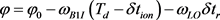(11)

${S}_{IFj}=\sqrt{2P}{D}_{j}\left(t-{t}_{s}\right){C}_{j}\left(t-{t}_{s}\right)\mathrm{cos}\left[{\omega }_{IF}t+\phi \right]+{n}_{j}\left(t\right)$ (12)

${S}_{IF}=\underset{j=1}{\overset{N}{\sum }}{\left\{\sqrt{2P}{D}_{j}\left(t-{t}_{s}\right){C}_{j}\left(\left(1+\eta \right)\left(t-{t}_{s}\right)\right)\mathrm{cos}\left[\left({\omega }_{IF}-{\omega }_{d}\right)t+\phi \right]+{n}_{j}\left(t\right)\right\}}_{j}$ (13)

②仿真实现

BDS中频信号系统仿真流程如图3所示，主要包括以下功能模块：一是BDS导航电文仿真模块，基于BDS星历数据，依靠BDS-SIS-ICD-B1I-1.0文件定义的导航电文编码格式及误差模型，编制下行导航电文；二是信号状态参数设置模块，包含仿真时间、电离层延迟、对流层延迟等误差模型参数及中频信号参数的设置；三是BDS中频信号生成模块，根据仿真时间段内可视卫星数，仿真多颗BDS卫星合成信号。该中频信号仿真器为GPS、Galileo星座预留接口，依据相应的ICD文件就可以生成对应的导航电文，结合不同星座的载波频率就可以扩展为对应星座下的中频信号。Figure 3. IF signal simulation flow chart

$\begin{array}{l}\text{G1}\left(X\right)=1+X+{X}^{7}+{X}^{8}+{X}^{9}+{X}^{10}+{X}^{11}\\ \text{G}2\left(X\right)=1+X+{X}^{2}+{X}^{3}+{X}^{4}+{X}^{5}+{X}^{8}+{X}^{9}+{X}^{11}\end{array}$ (14)

$\left\{\begin{array}{ll}{V}_{径向}\le \text{100}\text{\hspace{0.17em}}\text{m}/\text{s}\hfill & {t}_{step}=1\text{\hspace{0.17em}}\text{ms}\hfill \\ 100\text{\hspace{0.17em}}\text{m}/\text{s}<{V}_{径向}\le \text{1000}\text{\hspace{0.17em}}\text{m}/\text{s}\hfill & {t}_{step}=100\text{\hspace{0.17em}}\mu \text{s}\hfill \\ \text{1000}\text{\hspace{0.17em}}\text{m}/\text{s}<{V}_{径向}\le \text{10000}\text{\hspace{0.17em}}\text{m}/\text{s}\hfill & {t}_{step}=10\text{\hspace{0.17em}}\mu \text{s}\hfill \end{array}$ (15)

BDS接收机基带信号处理算法参考GNSS软件接收机的设计思路，实现对BDS信号的捕获、跟踪、解调，环路估计等信息直接输入导航滤波器进行后续组合解算，这里不做详细介绍。

3.4. INS力学编排

INS力学编排是根据INS的观测信息选取适当的数学模型计算出载体的速度、位置及姿态信息等导航定位参数。具体为：利用陀螺仪测得的载体相对于惯性坐标系的旋转角速度，计算得到载体坐标系至导航坐标系的转换矩阵；利用转换矩阵将加速度计测得的载体相对于惯性空间的加速度转换到导航坐标系，并补偿掉重力和Coriolis加速度，经积分得到导航定位信息  。相应的数据流程如图4所示。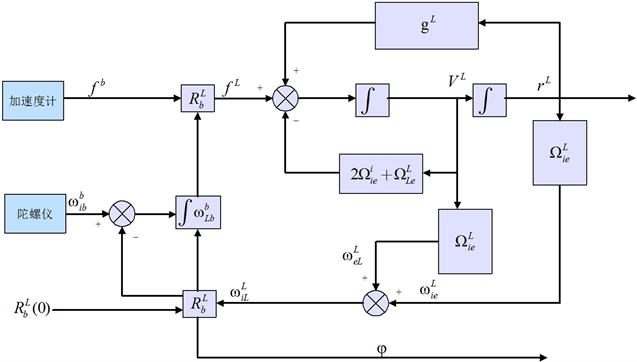Figure 4. Flow chart of mechanical orchestration data in the local horizontal coordinate system

${\stackrel{˙}{X}}^{L}=\left[\begin{array}{c}{\stackrel{˙}{r}}^{L}\\ {\stackrel{˙}{V}}^{L}\\ {\stackrel{˙}{R}}_{b}^{L}\end{array}\right]=\left[\begin{array}{c}{D}^{-1}{V}^{L}\\ {R}_{b}^{L}{f}^{b}-\left(2{\Omega }_{ie}^{L}+{\Omega }_{eL}^{L}\right){V}^{L}+{g}^{L}\\ {R}_{b}^{L}\left({\Omega }_{ib}^{b}-{\Omega }_{iL}^{b}\right)\end{array}\right]$ (16)

4. 性能仿真分析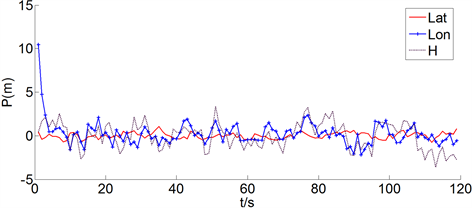Figure 5. Position error curve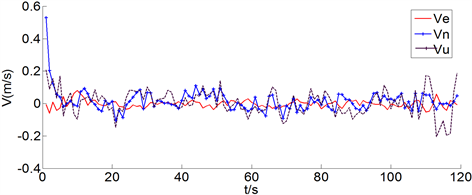Figure 6. Speed error curve

1、在高动态条件下，BDS/INS深组合导航系统的位置、速度误差迅速收敛稳定在很小的误差范围内。位置方向的误差稳定在4 m范围内，速度误差稳定在0.2 m/s范围内。

2、经度方向位置误差及东向速度误差在仿真时出现较大偏差，是由于仿真时载体可观测性较差。

5. 结束语

BDS/INS深组合导航具有广阔的应用前景，是目前研究的重点和热点。进行物理和半物理仿真试验，代价昂贵且试验难度大，而构建全数字仿真平台可以建立和提供接近实际的物理模型和工作参数，从而为方案论证提供依据，经济性和适应性强。本文给出BDS/INS深组合导航仿真平台的设计及实现方法，对系仿真平台的体系结构及信息流程进行详细的介绍。并以此平台为基础进行仿真分析，结果表明，高动态应用背景下，BDS/MEMS IMU深组合导航系统的位置误差稳定在4 m范围内，速度误差稳定在0.2 m/s内，这也表明了本文构建的BDS/INS深组合导航平台的正确性及有效性。后续将开展干扰情况下BDS/INS深组合导航精度相关研究，本研究内容对下一步设计BDS/INS深组合导航样机具有指导意义。

Study of Simulation Platform for BDS/INS Deep Integration Navigation[J]. 传感器技术与应用, 2019, 07(02): 66-73. https://doi.org/10.12677/JSTA.2019.72008

1. 1. 贾超广, 肖海霞. 基于卫星信号模拟器的北斗接收机性能测试与分析[J]. 导航定位学报, 2013, 1(4): 14-17.

2. 2. 唐康华, 吴美平, 胡小平. MEMS IMU辅助的高性能GPS接收机设计[J]. 测绘学报, 2008, 37(1): 128-134.

3. 3. 高鹏, 闫立伟, 富立, 王玲玲. 一种微惯性/软件接收机超紧组合方案研究[C]//中国科学院高技术研究与发展局, 中国卫星导航定位应用管理中心. 第一届中国卫星导航学术年会论文集. 2010: 316-323.

4. 4. Jovancevic, A., Brown, A., Ganguly, S., Noronha, J. and Sirpatil, B. (2004) Ultra Tight Coupling Implementation Using Real Time Software Receiver. Proceedings of ION GNSS 2004, Long Beach, 21-24 September 2004, 1575-1586.

5. 5. Babu, R. and Wang, J. (2005) Analysis of INS Derived Doppler Effects on Carrier Tracking Loop. The Journal of Navigation, 58, 493-507. https://doi.org/10.1017/S0373463305003309

6. 6. Babu, R. and Wang, J. (2006) Ul-tra-Tight Integration of Pseudolites with INS. Proceedings of IEEE/ION PLANS 2006, San Diego, 25-27 April 2006, 705-713.

7. 7. Sun, D. (2010) Ultra-Tight GPS/Reduced IMU for Land Vehicle Navigation. Canada UCGE Reports 20305, the Uni-versity of Calgary, Calgary.

8. 8. Woessner, W. and Noronha, J. (2006) A Software Defined Real-Time Ultra-Tightly Coupled GNSS-INS Architecture. ION GNSS 19th International Technical Meeting of the Satellite Division, Fort Worth, 26-29 September 2006, 2695-2703.

9. 9. 何晓峰. 北斗/微惯性组合导航方法研究[D]: [博士学位论文]. 长沙: 国防科学技术大学, 2009.

10. 10. 祖秉法. “北斗二号”民用软件接收机关键技术研究[D]: [博士学位论文]. 哈尔滨: 哈尔滨工程大学, 2010.

11. 11. 董俊杰. 基于Matlab的北斗二代B1频点软件接收机研究与实现[D]: [硕士学位论文]. 西安: 长安大学, 2014.

12. 12. 罗正平. 北斗二号民用软件接收机的算法研究与仿真[D]: [硕士学位论文]. 成都: 电子科技大学, 2012.

13. 13. 陈坡. GNSS/INS深组合导航理论与方法研究[D]: [博士学位论文]. 郑州: 解放军信息工程大学, 2013.

14. 14. 文立, 李荣冰, 刘建业. 基于北斗二代的多导航星座的惯性深组合算法研究[C]//中国科学院高技术研究与发展局, 中国卫星导航定位应用管理中心. 第一届中国卫星导航学术年会论文集.

15. 15. 王新龙, 于洁. SINS辅助GPS软件接收机的设计与实现[C]//中国科学院高技术研究与发展局, 中国卫星导航定位应用管理中心. 第一届中国卫星导航学术年会论文集.

16. 16. 中国卫星导航系统管理办公室. 北斗卫星导航系统公开服务性能规范(1.0版). 2013.

17. 17. 中国卫星导航系统管理办公室. 北斗卫星导航系统空间信号接口控制文件(1.0版). 2012.